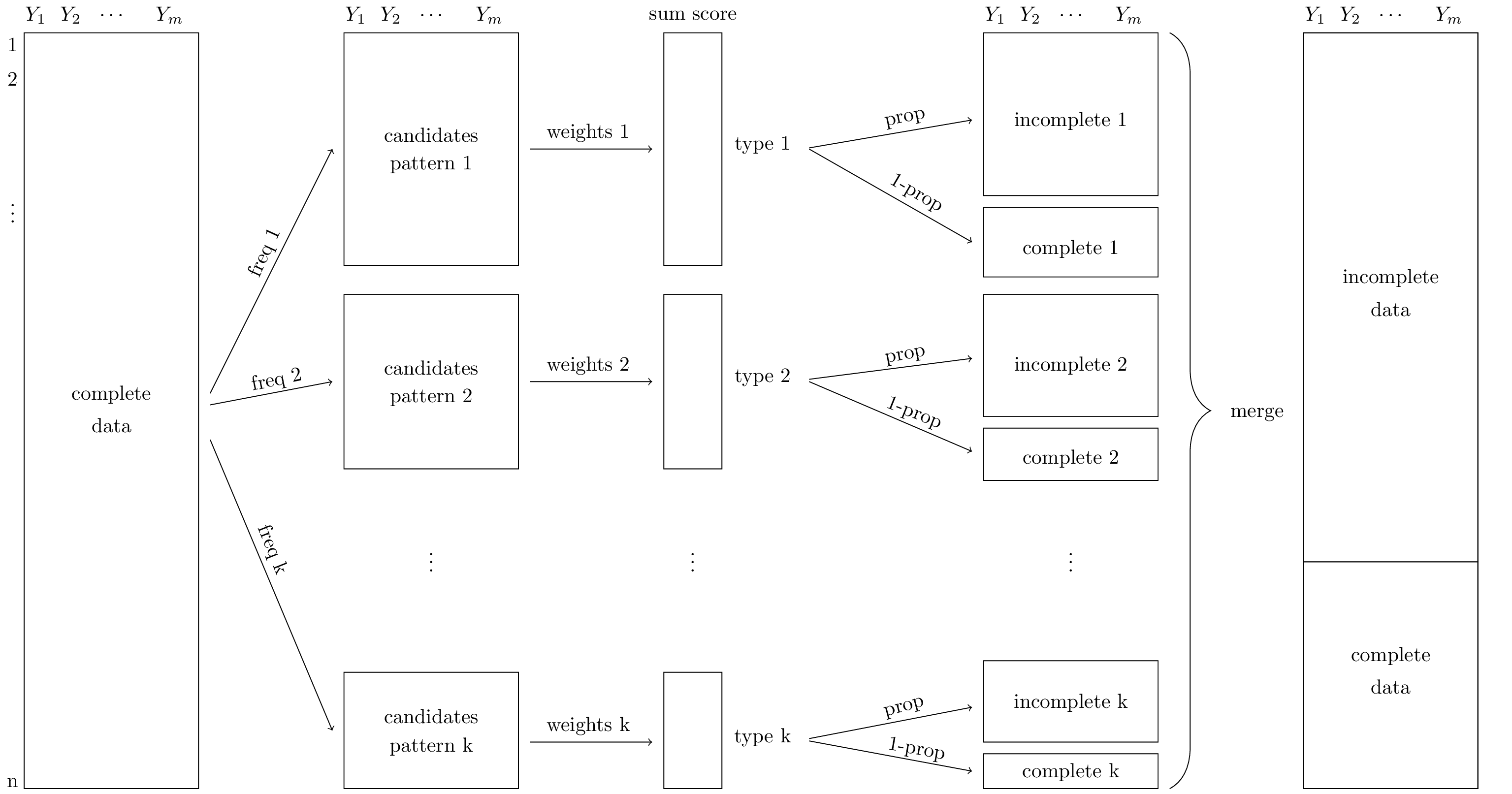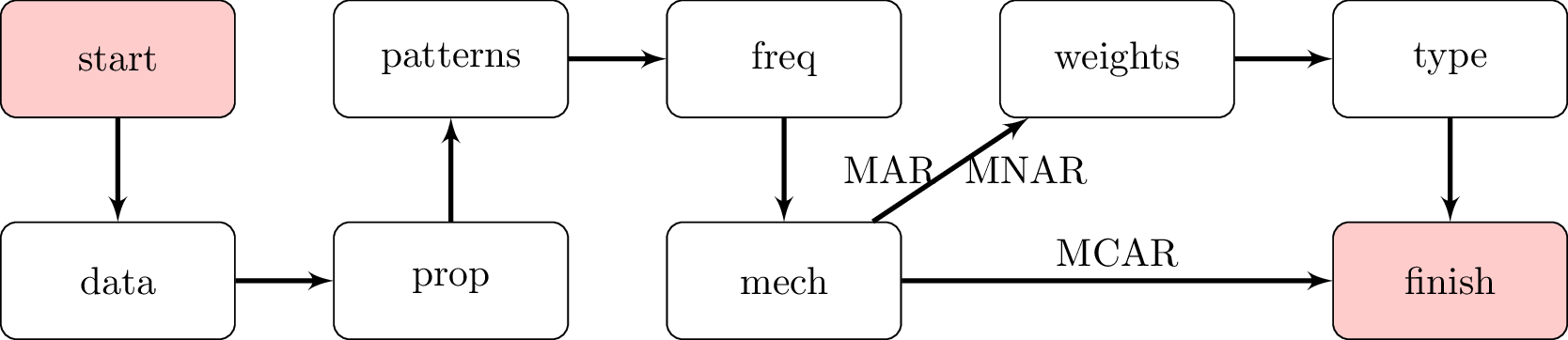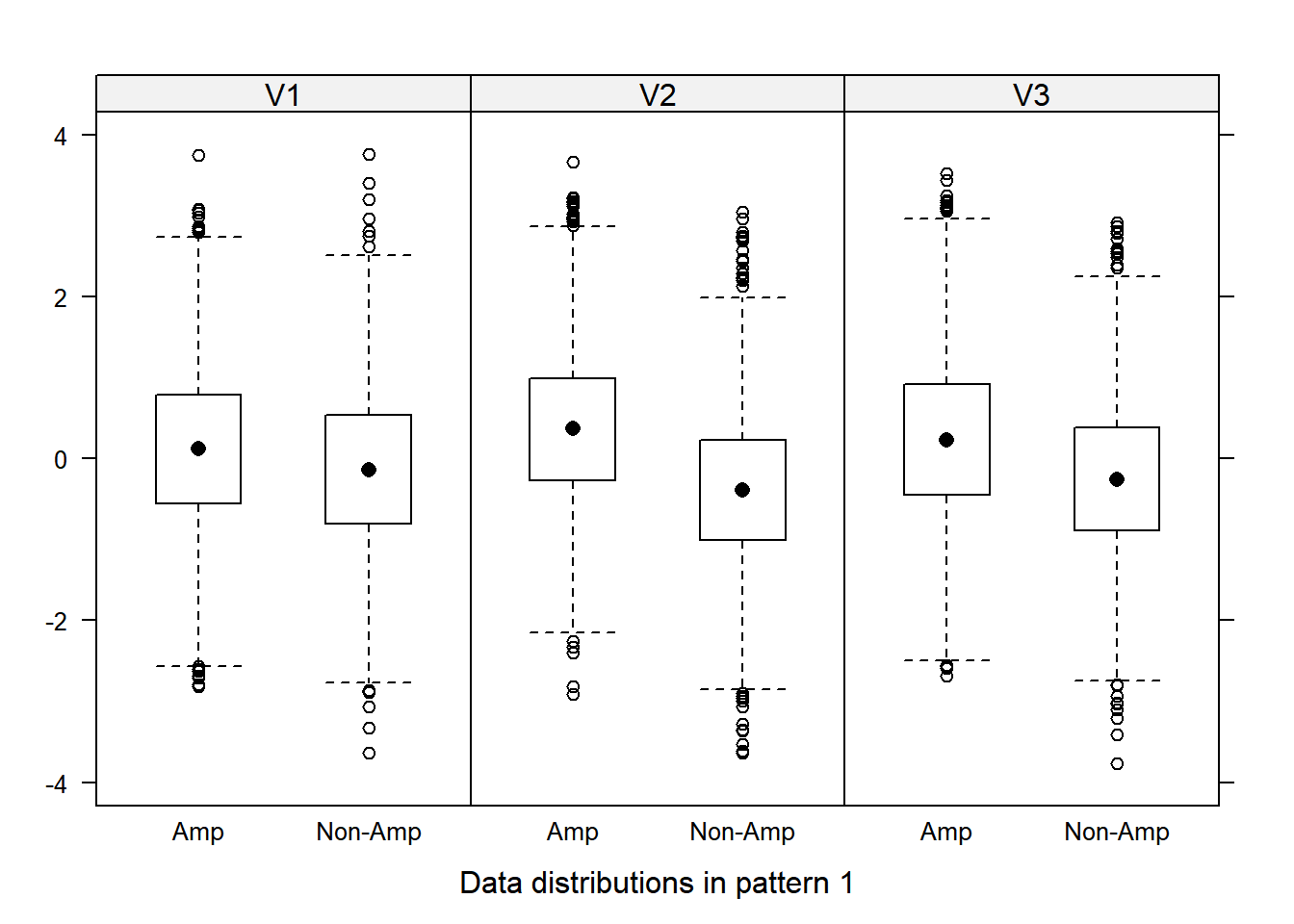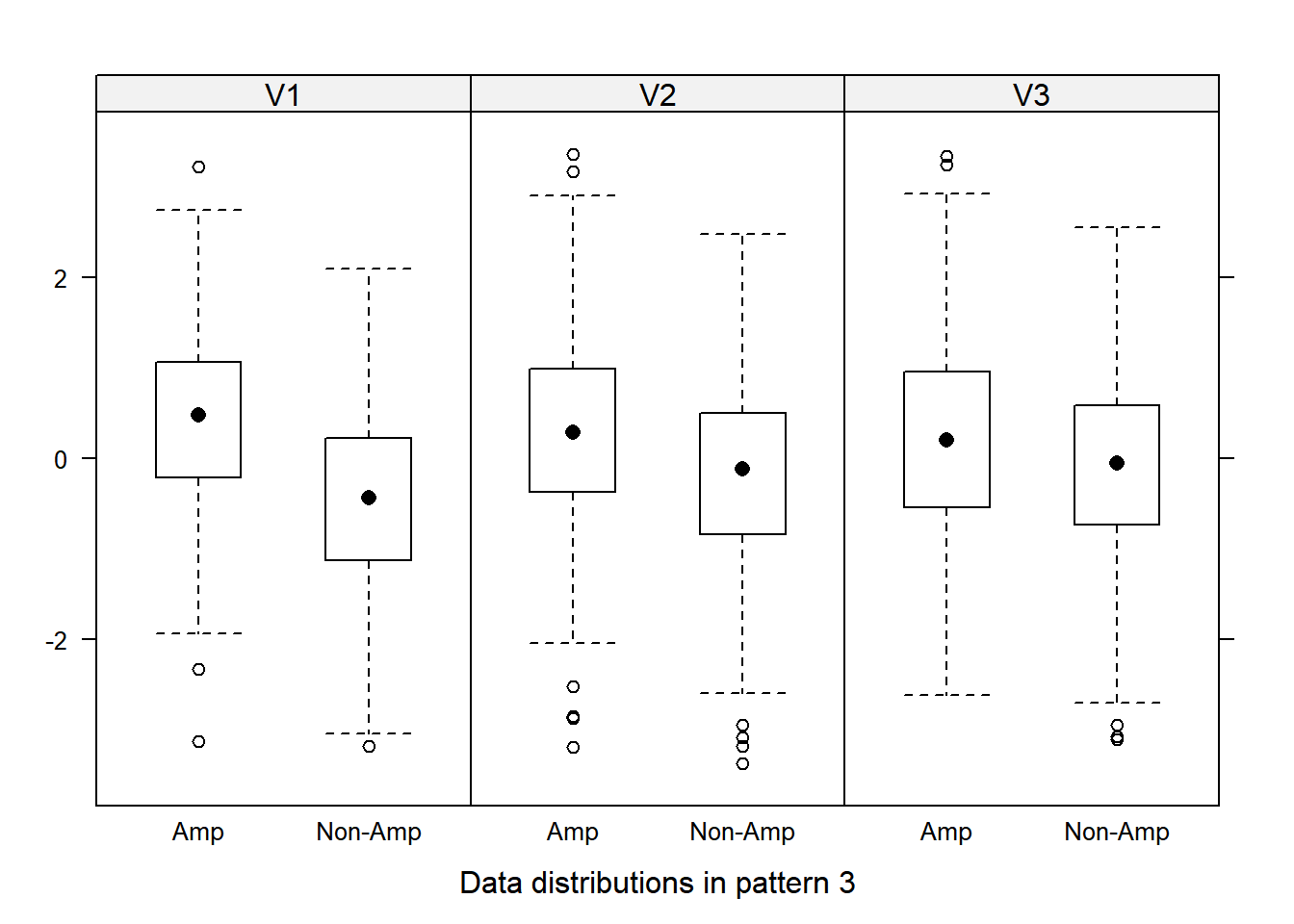### mice::ampute

We present a method to accurately evaluate the effect of missing data on statistical inferences. R-function ampute is an easy-to-use implementation of a multivariate missing data generation procedure. With ampute, it is straightforward to generate missing values in multiple variables, with different missing data proportions and varying underlying missingness mechanisms.

ampute is especially useful for the evaluation of missing data methods, but can also be used for the creation of planned missing data designs, for examination of the effect of measurement error on statistical inferences and for research in the field of multiple source data.

In general, a missing data methodology is evaluated by means of simulations. Such simulation studies generally have four steps:

1. A multivariate, complete data set is simulated and considered the population of interest.
2. This data set is then made incomplete.
3. The incomplete data are estimated by means of the missing data method.
4. Statistical inferences are obtained for the original, complete data set and after dealing with the missing values. A comparison of these inferences gives an indication of the performance of the missing data method.

An important aspect of these studies is the generation of missing values in a simulated, complete data set: the amputation procedure.

We demonstrate the performance of ampute and its improvement over the current amputation practice in our article Generating missing values for simulation purposes: A multivariate amputation procedure. In this article, we describe that the current amputation practice may not be appropriate for the generation of intuitive and reliable missing data problems. That is to say, important missing data characteristics such as the missingness percentage and the impact on statistical estimates influence each other. On the other hand, we demonstrate that the multivariate amputation procedure generates reliable amputations and allows for a proper regulation of missing data problems. The procedure has additional features to generate any missing data scenario precisely as intended. Hence, the multivariate amputation procedure is an efficient method to accurately evaluate missing data methodology.

ampute is available in multiple imputation package mice.

In this vignette we will:

1. give a concise summary of the missing data generation procedure
2. explain how to use ampute to make sophisticated missingness
3. discuss some additional features of ampute

### 1. The multivariate amputation procedure

The multivariate amputation procedure of ampute is built upon an initial idea proposed by Brand (1999). Figure 1 shows a schematic overview of the resulting amputation procedure. The method requires a complete dataset of $$n$$ participants and $$m$$ variables. The result of the procedure consists of multiple subsets with either incomplete or complete data. These subsets are merged to obtain an incomplete version of the original dataset.The amputation procedure starts with the user deciding what kinds of missing data patterns he desires to establish. A missing data pattern is a particular combination of variables with missing values and variables remaining complete. Based on the number of missing data patterns $$k$$,the complete dataset is randomly divided into $$k$$ subsets. The size of these subsets may differ between the patterns.

For MCAR missingnes, the next step involves the specification of the missingness proportion. For MAR and MNAR missingness, we calculate so-called weighted sum scores. A weighted sum score is simply the outcome of a linear regression equation where the coefficients are determined by the user. Based on the coefficients (i.e. weights) and a candidate’s variable value, each candidate obtains a different weighted sum score. Every variable may or may not play a role in this calculation.

The third step of the procedure comprehends the allocation of probabilities. For MCAR missingness, this probability is fixed and is determined by the missingness proportion. For MAR and MNAR missingness, a candidate obtains a probability of being missing based on his weighted sum score. The relation between the weighted sum scores and the probabilities are determined by one of four possible logistic distribution functions. For instance, cases with high weighted sum scores might have a higher probability of being missing than cases with low weighted sum scores. In the end, the allocated probabilities are executed. As a result, the candidates are divided into two groups: one group will receive the missing data pattern of its candidacy while the other group remains complete. Logically, the number of candidates who will receive missing values depends on the desired missingness proportion.

Although all these steps are connected, ampute provides a way to vary the missing data generation procedure without influencing other parameters. Therefore we developed ampute such that each step of the procedure can be manipulated with one of the function’s arguments. Figure 2 shows the most important arguments.### 2. ampute’s arguments

ampute contains several arguments to manipulate the features of the generated missing dat problem. We will now continue with a more thorough explanation of each of the arguments from Figure 2. In short, the arguments are used for the following: 1) data: feed function complete data 2) prop: define missingness proportion 3) patterns: specify missing data patterns 4) freq: specify relative occurrence of these patterns 5) mech: choose between MCAR, MAR and MNAR mechanisms 6) weights: specify weights for calculation of weighted sum scores 7) type: choose RIGHT, MID, TAIL or LEFT logistic distribution function

require("mice")

#### 2.1 Data

The first argument is an input argument for a complete dataset. In simulation settings, multivariate data can be generated by using function mvrnorm from R-package MASS. Be aware that the covariance matrix should be semi definite.

set.seed(2016)
testdata <- MASS::mvrnorm(n = 10000, mu = c(10, 5, 0), Sigma = matrix(data = c(1.0, 0.2, 0.2, 0.2, 1.0, 0.2, 0.2, 0.2, 1.0), nrow = 3, byrow = T))
testdata <- as.data.frame(testdata)
summary(testdata)
##        V1               V2               V3
##  Min.   : 6.346   Min.   :0.9789   Min.   :-3.799124
##  1st Qu.: 9.317   1st Qu.:4.3199   1st Qu.:-0.690542
##  Median :10.003   Median :4.9963   Median :-0.009941
##  Mean   : 9.998   Mean   :4.9973   Mean   : 0.000759
##  3rd Qu.:10.677   3rd Qu.:5.6759   3rd Qu.: 0.673069
##  Max.   :13.770   Max.   :8.6579   Max.   : 3.810729

The amputation procedure can immediately be executed when this dataset is entered into the function. Storing the result allows you to work with the amputed data.

result <- ampute(testdata)
result
## Multivariate Amputed Data Set
## Call: ampute(data = testdata)
## Proportion of Missingness:  0.5
## Frequency of Patterns:  0.3333333 0.3333333 0.3333333
## Pattern Matrix:
##   V1 V2 V3
## 1  0  1  1
## 2  1  0  1
## 3  1  1  0
## Mechanism: "MAR"
## Weight Matrix:
##   V1 V2 V3
## 1  0  1  1
## 2  1  0  1
## 3  1  1  0
## Type Vector:
##  "RIGHT" "RIGHT" "RIGHT"
## Odds Matrix:
##      [,1] [,2] [,3] [,4]
## [1,]    1    2    3    4
## [2,]    1    2    3    4
## [3,]    1    2    3    4
## Head of Amputed Data Set
##          V1       V2         V3
## 1        NA 6.018999  0.3681981
## 2  9.668991 4.391821 -1.1127595
## 3 10.273415 4.662521  0.1796964
## 4 10.124800 4.055544         NA
## 5        NA 7.171578  1.7141378
## 6 10.803311 5.038649         NA

The incomplete dataset is stored under amp. To see whether the amputation has gone according plan, a quick investigation can be done by using function md.pattern.

md.pattern(result$amp) ## V3 V1 V2 ## 5014 1 1 1 0 ## 1670 1 0 1 1 ## 1670 1 1 0 1 ## 1646 0 1 1 1 ## 1646 1670 1670 4986 The rows of the table show the different missing data patterns with the number of cases accordingly. The first row always refers to the complete cases. The last column contains the number of variables with missing values in that specific pattern. Consequently, each column total describes the number of cells with missing values for that variable. A more thorough explanation of md.pattern can be found in its help file (?md.pattern). Note that because md.pattern sorts the columns in increasing amounts of missing information, the order of the variables is different from the order in the data. #### 2.2 Prop The first step in generating a missing data problem in complete data is always the specification of the missingness proportion. In ampute, we call this argument prop. As a default, the missingness proportion is 0.5: result$prop
##  0.5

This means that 50% of the cases will have missing values. It is easy to change this proportion by using the argument prop. One might also want to specify the percentage of missing cells. For this, the argument bycases should be FALSE.

result <- ampute(testdata, prop = 0.2, bycases = FALSE)
md.pattern(result$amp) ## V3 V1 V2 ## 4046 1 1 1 0 ## 1987 1 0 1 1 ## 1998 1 1 0 1 ## 1969 0 1 1 1 ## 1969 1987 1998 5954 An inspection of the result shows that the proportion of missing cells is approximately 20%, as requested (the data set contains 10000 * 3 = 30000 cells, in total, 6425 cells are made missing). ampute automatically calculates how these 6000 missing cells are divided among the patterns. As a result, the proportion of missing cases is: result$prop
##  0.6

#### 2.3 Patterns

The basic idea of ampute is the generation of missingness patterns. Each pattern is a combination of missingness on specific variables while other variables remain complete. For example, someone could have forgotten the last page of a questionnaire, resulting in missingness on a specific set of questions. Another missingness pattern could occur when someone is not willing to answer private questions. Or when a participant misses a wave in a longitudinal study. In other words, each pattern is a specific combination of missing and complete variables.

The default missingness patterns can by obtained by:

mypatterns <- result$patterns mypatterns ## V1 V2 V3 ## 1 0 1 1 ## 2 1 0 1 ## 3 1 1 0 In the patterns matrix, each row refers to a missing data pattern and each column to a variable. 0 is used for variables that should have missing values in a particular pattern. 1 is used otherwise. Here, three missing data patterns are specified with missing values on one variable only. Note that as a result of this, none of the cases will have missingness on more than one variable. A case either has missingness on V1, V2 or V3 or remains complete. It is possible to manipulate the matrix by changing the values or adding rows. For example, we can change the missingness patterns as follows: mypatterns[2, 1] <- 0 mypatterns <- rbind(mypatterns, c(0, 1, 0)) mypatterns ## V1 V2 V3 ## 1 0 1 1 ## 2 0 0 1 ## 3 1 1 0 ## 4 0 1 0 By doing this, we create a missingness pattern where cases will have missingness on V1 and V2 but not on V3 (pattern 2). Also, I have added a fourth missing data pattern to create a combinaton of missingness on V1 and V3. Now, I can perform the amputation again with the desired patterns matrix as its third argument. Inspect the result with the md.pattern function. result <- ampute(testdata, patterns = mypatterns) md.pattern(result$amp)
##        V2   V3   V1
## 5079    1    1    1    0
## 1216    1    1    0    1
## 1248    1    0    1    1
## 1257    0    1    0    2
## 1200    1    0    0    2
##      1257 2448 3673 7378

#### 2.4 Freq

The function ampute works by dividing the complete dataset into multiple subsets. The number of these subsets is determined by the number of patterns because all cases are divided over the subsets. As such, they become candidate for a certain missing data pattern.

The size of the subsets, and thereby the relative occurrence of the missing data pattern, can be determined with argument freq. This argument is a vector with values between 0 and 1. The number of values determines the number of subsets and must be equal to the number of patterns. The values themselves are interpreted relatively from each other.

For example,

result$freq ##  0.25 0.25 0.25 0.25 this frequency vector has four values of equal size. This means that four subsets with equal size are created. We can adapt the frequency vector such that subset one becomes much larger than the other subsets. For example: myfreq <- c(0.7, 0.1, 0.1, 0.1) Note that the sum of the frequency values should always be 1 in order to divide all the cases over the subsets. result <- ampute(testdata, freq = myfreq, patterns = mypatterns) md.pattern(result$amp)
##       V2  V3   V1
## 4982   1   1    1    0
## 3555   1   1    0    1
##  524   1   0    1    1
##  466   0   1    0    2
##  473   1   0    0    2
##      466 997 4494 5957

With md.pattern we can check whether the frequency specifications are performed as intended. It turns out there are indeed four missing data patterns with the first pattern occuring seven times as often as the other three patterns.

#### 2.5 Mech

At this point, we have to decide which kind of missingness mechanism we are going to implement. For more information about missingness mechanisms, I refer to Van Buuren (2012), but in short, we distinguish MCAR, MAR and MNAR missingness. With MCAR missingness, the missing values are implemented completely at random. With MAR missingness, the missingness depends on the values of observed variables. With MNAR missingness, the missingness depends on the missing values themselves.

Now, ampute’s argument mech is a string which needs either "MCAR", "MAR" or "MNAR". For MCAR missingness, only the argument prop needs another specification (or you can leave it at the default). With MAR and MNAR missingness, the arguments weights and type can be used to manipulate the characteristics of the missing data problem.

As a default mech == "MAR":

result$mech ##  "MAR" #### 2.6 Weights With this argument, we can determine how the values in the dataset are related to whether they become missing or not. After specifying the so-called weights matrix, we calculate a weighted sum score for each candidate. Before we explain how this calculation takes place, it is important to know that we will use the weighted sum scores to determine whether a case receives missing values or not. Namely, based on his weighted sum score, each candidate obtains a probability of being missing. For the allocation of these probabilities, we use logistic distribution functions. We will discuss these distributions function in part 2.6 of this vignette: type. Basically, the idea is that, for instance, cases with high weighted sum scores will have a higher probability of being missing than cases with low weighted sum scores. The weighted sum scores are built from the variable values and certain pre-specified weights. In fact, a weighted sum score is simply the outcome of a linear regression equation where the coefficients are determined by us. Thus, the weighted sum score of case $$i$$ is calculated as follows: $\begin{equation*} wss_i = w_1 \cdot y_{1i} + w_2 \cdot y_{2i} + ... + w_m \cdot y_{mi}, \end{equation*}$ where $$\{y_{1i}, y_{2i}, ..., y_{mi}\}$$ is the set of variable values of case $$i$$ and $$\{w_1, w_2, ..., w_m\}$$ are the corresponding pre-specified weights. For our example, $$j\in\{1, 2, 3\}$$ and $$k\in\{1, 2, 3, 4\}$$ because there are three variables and four missing data patterns. For every pattern we can set one weight for every variable to govern the impact of the variables on the formation of the sum score. Variables with higher weights will have a larger influence on the size of the weighted sum score than variables with lower weights. For instance, if variables V1 and V2 have weight values 4 and 2 respectively, V1’s importance is twice as large as that of V2. Note that the influence of the weights is relative; in the example above, weight values of 0.4 and 0.2 would have an equivalent effect on the calculation of the weighted sum scores. The sign of the weight values influences whether a weighted sum score increases or decreases. Namely, a positive weight will increase the weighted sum score while a negative weight will have a decreasing impact. Furthermore, each pattern can obtain its own weight values. For example, variable V1 can have a weight value of 4 in the first pattern, but a weight value of -2 in the second pattern. All the weights values are stored and used in R-function ampute by means of the weights matrix. This matrix has dimensions #patterns by #variables. The default weights matrix with a MAR missingness mechanism is as follows: result$weights
##   V1 V2 V3
## 1  0  1  1
## 2  0  0  1
## 3  1  1  0
## 4  0  1  0

Since we have four patterns, the weights matrix also contains four rows. Each of these rows contains a weight for every variable. In this situation, only weight values of 0 and 1 are used. This means that some variables are non-weighted. The variables that are weighted, are of equal importance (since they all have value 1). Of course, the idea of the weights matrix is to weight variables differently from each other. For instance, we can give variable V2 a higher weight than variable V3:

myweights <- result$weights myweights[1, ] <- c(0, 0.8, 0.4) By choosing the values 0.8 and 0.4, variable V2 is weighted twice as heavy as variable V3. For pattern 3, we will weight variable V1 three times as heavy as variable V2. myweights[3, ] <- c(3, 1, 0) myweights ## V1 V2 V3 ## 1 0 0.8 0.4 ## 2 0 0.0 1.0 ## 3 3 1.0 0.0 ## 4 0 1.0 0.0 Before we continue to apply these weights, we must remark that an important feature of the multivariate amputation procedure is situated in the possibility to choose a weight value of zero. A zero weight indicates that the values of that variable play no role in the calculation of the weighted sum scores. Since the probabilities of being missing are based on the weighted sum scores, non-weighted variables will become independent in the process of determining which cases will obtain missing values. Since, by definition, MAR missingness means that the probability of being missing depends on the values of observed variables, we can generate a MAR missingness mechanism by assigning zero weights to all variables that will be amputed. In contrast, if we desire to give a non-zero weight to one or more of the variables that will be amputed, the generated missingness mechanism is MNAR. This effect is easy to see if you compare the default weights matrix with our patterns matrix in situation of a MNAR missingness mechanism. result <- ampute(testdata, freq = myfreq, patterns = mypatterns, mech = "MNAR") result$patterns
##   V1 V2 V3
## 1  0  1  1
## 2  0  0  1
## 3  1  1  0
## 4  0  1  0
result$weights ## V1 V2 V3 ## 1 1 0 0 ## 2 1 1 0 ## 3 0 0 1 ## 4 1 0 1 In the patterns matrix, the variables that will be amputed are coded with 0. In the weights matrix, these exact same variables are weighted with 1. Apparently, the variables that will be made incomplete are determining which cases will be made missing. With MAR missingness, this is exactly opposite. Of course, if you create your own weights matrix, you can make nice variants and combinations of MAR and MNAR missingness. We will now apply our patterns and weights matrices and inspect the results in two ways: boxplots and scatterplots. result <- ampute(testdata, freq = myfreq, patterns = mypatterns, weights = myweights) ##### Boxplots Within pacakge mice, we developed function bwplot to easily see the distributions of the amputed and non-amputed data. This plot function might be useful because the boxplots show the relation between the missingness and the variables values. With function bwplot, argument which.pat can be used to specify the patterns you are interested in (default: all patterns). The argument yvar should contain the variables names (default: all variables). Besides, the function returns the mean, variance and $$n$$ of the amputed and non-amputed data for each variable and each pattern requested. In the column Amp, a 1 refers to the amputed data and 0 to the non-amputed data. If descriptives are not required, the argument descriptives can be set to FALSE. bwplot(result, which.pat = c(1, 3), descriptives = TRUE) ##$Descriptives
## , , Variable =  V1
##
##        Descriptives
## Pattern Amp     Mean     Var    N
##       1   1  0.11770 0.96079 3552
##       1   0 -0.12512 0.99905 3495
##       3   1  0.44272 0.82890  496
##       3   0 -0.45347 0.91124  488
##
## , , Variable =  V2
##
##        Descriptives
## Pattern Amp     Mean     Var    N
##       1   1  0.36608 0.84028 3552
##       1   0 -0.39542 0.85435 3495
##       3   1  0.30720 1.02448  496
##       3   0 -0.15719 1.02524  488
##
## , , Variable =  V3
##
##        Descriptives
## Pattern Amp     Mean     Var    N
##       1   1  0.22595 0.96457 3552
##       1   0 -0.26021 0.90809 3495
##       3   1  0.22467 1.05373  496
##       3   0 -0.09248 0.99571  488
##
##
## $Boxplot pattern 1## ##$Boxplot pattern 3The medians and boundaries of the boxes show that in pattern 1, the amputed data are shifted to the right with respect to the non-amputed data. For variable V2, this effect is the largest, due to the weight value that was specified (0.8). For V1, there is a very small difference between the boxplots of the amputed and non-amputed data. This makes sense, because variable V1 was amputed in the first pattern and therefore set to 0 in the weights matrix. The small difference that is visible is due to the positive correlation between V1 on the one side and V2 and V3 on the other side. These correlations were created during the simulation of the data.

If desired, one could use the function tsum.test() from package BSDA to perform a t-test on the amputed and non-amputed data. The data returned in the descriptives table can be used for that. For example, to know whether the mean difference between the amputed and non-amputed data for variable V2 in pattern 1 is significant, one could run:

BSDA::tsum.test(mean.x = 0.39077, mean.y = -0.38992, s.x = sqrt(0.83774), s.y = sqrt(0.87721), n.x = 3473, n.y = 3493)
##
##  Welch Modified Two-Sample t-Test
##
## data:  Summarized x and y
## t = 35.184, df = 6961.9, p-value < 2.2e-16
## alternative hypothesis: true difference in means is not equal to 0
## 95 percent confidence interval:
##  0.7371929 0.8241871
## sample estimates:
## mean of x mean of y
##   0.39077  -0.38992

As is visible, there is a significant difference between the amputed and non-amputed data of variable V2 in pattern 1. For pattern 3, the difference between the distributions of the amputed and non-amputed data is largest for variable V1, as can be expected due to the weight values in pattern 3.

##### Scatterplots

Scatterplots also help to investigate the effect of the specifications. We can directly impose the function xyplot on the mads object. The function contains arguments comparable to bwplot. For example, we can investigate the weighted sum scores of pattern 1 as follows:

xyplot(result, which.pat = 1)
## \$Scatterplot Pattern 1